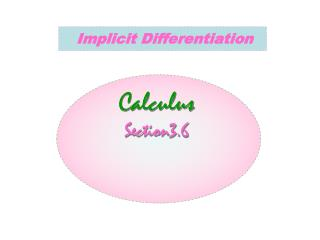Download PresentationImplicit Differentiation

# Implicit Differentiation - PowerPoint PPT Presentation

Implicit Differentiation. Calculus Section3.6. Chap.3.6 Implicit Differentiation -. Example 1 Differentiating Implicitly Find dy / dx if y 2 = x . Solution The equation y 2 = x defines two differentiable functions of x , namely. Chap.3.6 Implicit Differentiation -.I am the owner, or an agent authorized to act on behalf of the owner, of the copyrighted work described.
Download Presentation## Implicit Differentiation

An Image/Link below is provided (as is) to download presentation

Download Policy: Content on the Website is provided to you AS IS for your information and personal use and may not be sold / licensed / shared on other websites without getting consent from its author.While downloading, if for some reason you are not able to download a presentation, the publisher may have deleted the file from their server.

- - - - - - - - - - - - - - - - - - - - - - - - - - E N D - - - - - - - - - - - - - - - - - - - - - - - - - -
Presentation Transcript
1. Implicit Differentiation Calculus Section3.6

2. Chap.3.6 Implicit Differentiation - • Example 1 Differentiating Implicitly • Find dy/dx if y2 = x. Solution • The equation y2 = x defines two differentiable functions of x, namely

3. Chap.3.6 Implicit Differentiation - • To find dy/dx, we simply differentiate both sides of the equation y2 = x with respect to x, treating y = f(x) as a differentiable function of x :

4. This one formula gives the derivatives we calculated for both explicit solutions and

5. 3.6 Implicit Differentiation - Another example This is not a function, but it would still be nice to be able to find the slope. Do the same thing to both sides. Note use of chain rule.

6. Chap.3.6 Implicit Differentiation -VI • Example 2 Differentiating Implicitly • Find dy/dx if y2 = x2 + sin xy • Solution

7. The graph of y2 = x2 + sin xy in Example 2. The example shows how to find slopes on this implicitly defined curve.

8. Notice that the formula for dy/dx applies everywhere that the implicitly defined curve has a slope. Notice also that the derivative involves both variables x and y, not just the independent variable x.

9. Implicit Differentiation Find equations for the tangent and normal to the curve at (2, 4). Find the slope of the tangent at (2,4) find the slope of the normal (perpendicular) at (2,4) Sometimes called Orthogonal

10. Differentiate both sides of the equation with respect to x, treating y as a function of x. This requires the chain rule. • Collect terms with dy/dx on one side of the equation. • Factor dy/dx • 4. Solve for dy/dx

11. 1 Differentiate both sides w.r.t. x. 2 Solve for . 3.6 Implicit Differentiation Example 2 This can’t be solved for y. This is using implicit differentiation.

12. We need the slope. Since we can’t solve for y, we use implicit differentiation to solve for . 3.6 Implicit Differentiation Example 3 Find the equations of the lines tangent and normal to this curve at the indicated point Take the derivative of each term wrtx (with respect to x) Note product rule.

13. 3.6 Implicit Differentiation Example 2 cont’d a) To find the equation of the line tangent to this curve at the indicated point Sub in the values from the point

14. 3.6 Implicit Differentiation Example 2 cont’d a) To find the equation of the line tangent to the curve at the indicated point Use the point-slope formula

15. b) Find the equations of the lines tangent and normal to the curve at . tangent: normal:

16. 3.6 Implicit Differentiation Ex1 cont’d If y is a function of x then its derivative is If y2 is a function of y, which in turn is a function of x. Using the chain rule: Find the following derivatives wrt x

17. 3.6 Implicit Differentiation Ex. 1 cont’d. Use product rule

18. Another Example - Find dy/dx 1. Write the equation of the tangent line at (0,1)

19. Differentiate both sides of the equation with respect to x, treating y as a function of x. This requires the chain rule. • Collect terms with dy/dx on one side of the equation. • Factor dy/dx • 4. Solve for dy/dx

20. Find dy/dx 1. Write the equation of the tangent line at (0,1).Sub the values of x and y into dy/dx to find m

21. Find if . Substitute back into the equation. Higher Order Derivatives

22. Find if : 1) Solution

23. 2) Solution

24. Show that p(2,4) lies on the curve : Then find the eqs. Of the tangent and normal Lines at this point . Solution P(2,4) Graph. Now

25. Tangent line: Normal line:

26. Find if Where y 0 Solution

27. s.T